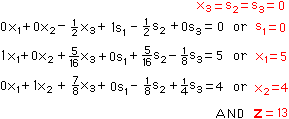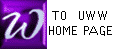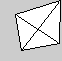a Simplex
EXAMPLE OF SIMPLEX PROCEDURE
FOR A STANDARD
LINEAR PROGRAMMING PROBLEM

 Below is the original problem: objective function is in green.
 Red variables below are called SLACK VARIABLES
 Below is the SIMPLEX TABLEAU. Compare RED symbols with Z = x1 + 2x2 - x3. Highlighted below is the "ISM".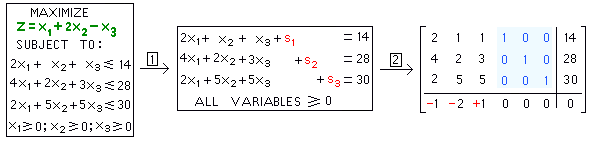The 1st SIMPLEX TABLEAU is below. (credit Steve Hulet) Note missing z-column Highlighted is the "ISM"
See steps 3,4,5 of
SIMPLEX METHOD
as you handle INDICATORS,
RATIOS, and PIVOTS.
 Named below are the 4 row operations needed to pivot on the number "5" encircled in red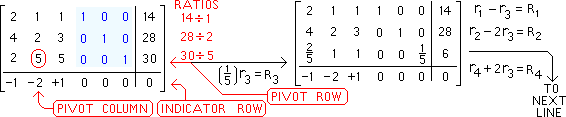Below are the results of therow operations named above highlighted is the new ISM
 Since one INDICATOR (namely -1/5) remains negative, we must repeat steps 3-9
 Below is the result of changing our pivot to "1"
 Named below are 4 row operations needed to pivot on the number(16/5) encircled in red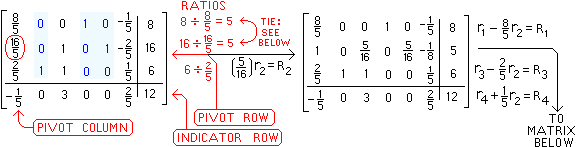Above there was a tie for least non-negative ratio: either row 1 or row 2 could have become the pivot row, and either choice leads to the final tableau after one additional pivoting. At the right is the result of the final 3 row operations. "ISM" is highlighted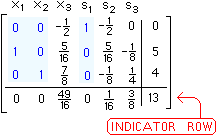All indicators {0, 0, 4916 , 0, 1 16 and 38 } are now zero or bigger ("13" is NOT an indicator). Thus, as in step 8 of the SIMPLEX METHOD, the last tableau is a FINAL TABLEAU. Row operations of SIMPLEX METHOD are done.
 Thus, the basic solution for the tableau above is the solution to our original problem. [1st] set equal to 0 all variables NOT associated with the above highlighted ISM. The columns of the final tableau have variable tags. [2nd] convert each row of the final tableau (except the bottom row) back into equation form (as at the right) to find the values of the remaining variables. The value of the objective function is in the lower right corner of the final tableau.